# DAV Class 4 Maths Chapter 5 Worksheet 4 Solutions

The DAV Class 4 Maths Book Solutions and DAV Class 4 Maths Chapter 5 Worksheet 4 Solutions of Length offer comprehensive answers to textbook questions.

## DAV Class 4 Maths Ch 5 WS 4 Solutions

Question 1.
Solve the following word problems.
(a) Neetu bought 3 m 75 cm of cloth for shirt and 2 m 20 cm of cloth for trouser. What is total length of cloth she bought?
Neetu bought cloth for shirt = 3m 75cm
Neetu bought cloth for trouser = 2m 20cm
Total length = 5m 95kmThus, total length of cloth is 5 m 95 cm.

(b) Amit travelled 15 km 550 m by train, 12 km 400 m by bus and 1 km 250 m by scooter. How much distance did he travel in all?
Amit travelled by train = 15km 550m
Amit travelled by bus = 12km 400 m
Amit travelled by scooter = 1km 250m
Total distance = 29km 200mThus, Amit travelled 29 km 200 m in all.

(c) Mrs. Renu has a ribbon 16 m 75 cm long. She cuts it into two pieces. One piece is 8 m 90 cm. Find the length of the other piece.
Total length of ribbon = 16m 75cm
Length of one piece of ribbon = 8m 90cm
Length of other piece of ribbon = 7m 85cm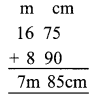Thus, the length of other piece is 7 m 85 cm.

(d) The heights of Ram and Shyam are 1 m 75 cm and 1 m 28 cm respectively. Who is taller and by how much?
Height of Ram = 1 m 75
Height of Shyam = 1 m 28 cm.
1 m 75 cm > 1 m 28 cm m cm
∴ Height of Ram = 1 m 75 cm
Height of Shyam = 1m 28 cm
Comparison = 0 m 47 cm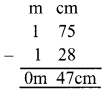Thus, Ram is 47 cm taller than Shyam.

(e) An ant climbed 9 m 50 cm on a wall. Then it came down 4 m 75 cm along. The same wall. How far is the ant from the starting point?
Ant climbed a distance on wall = 9m 50 cm
Ant came down = – 4m 75cm
Distance from starting point = 4m 75cm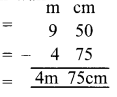Thus, the ant is 4 m 75 cm away from the starting point.

### DAV Class 4 Maths Chapter 5 Value Based Questions

Question 1.
How much distance will Amit’s father travel now for dropping all the children to school?
Distance travel in dropping Amit = More Distance travel in dropping three childrenThus, 4 m 75 cm away from the starting point.

Question 2.
What are the advantages of car pooling?
Car pooling saves fuel and also environment from getting polluted.

Word Problems

E.g.1.
Mala needs a garland of 12 m 67 cm for decoration of door and 7 m 15 cm for window. What is the total length of garland needed by Mala.
We have to add the length of garland required to decorate door and window to get total length of garland.
Length of garland for door = 12m 67 cm
Length of garland for window = 7 m 15 cm
Total length = 19m 82cmE.g.2.
Meeta bought 75 m 60 cm cloth roll. If 58 m 70 cm of the cloth is used for making curtain for doors and windows, how much cloth is left on the roll?
We have to subtract the length of cloth used for making curtains from the cloth roll.
Total length of cloth = 75m 60 cm
Cloth used in curtains = – 58m 70 cm
Cloth left = 16m 90cm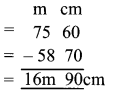Question 1.
Convert each of the following into metres:
(a) 208 km
208 km
= 208 x 1000m
= 208000 m

(b) 1 km 25 m
1 km 25 m
= 1 × 1000m + 25m
= 1025 m

(c) 4 km 65
4 km 65m
= 4 × 1000m 65m
= 4065 m

(d) 5 km 30 m
5km 30m
= 5 × 1000m + 30 m
= 5030 m

(e) 10 km 165 m
10 km 165 m
= 10 × 1000 m + 165 m
= 10165 mQuestion 2.
(a) Convert 600 cm into m and cm.
(600 + 100) meters (1cm = $$\frac{1}{100}$$m)
= 6 metres.

(b) Convert 748 cm into m and cm.
(748 100) meters (1cm = $$\frac{1}{100}$$m)
= 7 m 48 cm.

(c) Convert 546 cm into m and cm.
(546 100) meters (1cm = $$\frac{1}{100}$$m)
= 5 m 46 cm.

Question 3.
Convert the following kilometres into metres:
(i) 740 km
740 × 1000 metres (1 km = 1000 m)
= 740000 metres.

(ii) 71 km
71 × 1000 metres (1km = 1000 m)
= 71000 metres

(iii) 405 km
405 × 1000 metres (1km = 1000 m)
= 405000 metresQuestion 4.
(i) 17 m 50 cm and 15 m 20 cm.32 m 70 cm

(ii) 72 km 350 m and 43 km 790 m.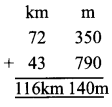116 km 140 m

(iii) 26 m 20 cm and 36 m 50 cm.62 m 70 cm

(iv) 35 km 150 m and 43 km 620 m.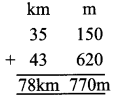78 km 770 m

Question 5.
Subtract:
(a) 10 m 57 cm and 4 m 96 cm.5m 61cm

(b) 7 m 80 cm and 3 m 95 cm.3m 85cm

(c) 6 km 300 m and 2 km 120 m.4km 180m

(d) 47 km 690 m and 34 km 695 m.